GeeksforGeeks App
Open AppBrowser
Continue

# OpenCV | Understanding Brightness in an Image

In OpenCV, changing the brightness of an image is a very basic task to perform. By changing the image brightness, it is meant to change the value of each and every image pixel. This change can be done by either increasing or decreasing the pixel values of the image, by any constant.

To increase the brightness levels of the image, simply add a constant positive value to each and every image pixel.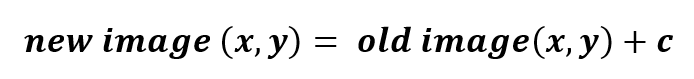Similarly if one wishes to decrease the brightness level of the image, then subtract a constant positive value for each and every image pixel.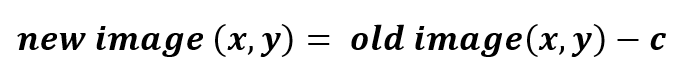Let’s take an assumption by considering the below-mentioned matrix as our image matrix with values of pixel ranging from 0 to 255 and let’s assume that the data type for this image is CV_8UC3

## Increasing Brightness :

So. let’s suppose we wish to increase image brightness by a value of 60. Then we add 60 to each image pixel value but it is strictly forbidden to exceed the pixel range i.e. the allowed maximum limit is 255 and our pixel value should not increase 255. Suppose if in any case, this image pixel value exceeds 255, then it should be given the maximum allowed value only instead of the newly increased value.

Input :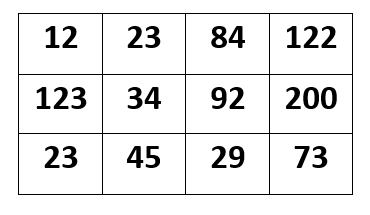Output (After increasing the image pixel value by 60):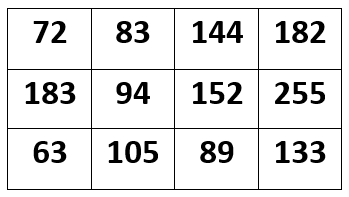Let’s check the image matrix given below, we have increased this value by 60 but the pixel value “255” has not been increased to 260 as we add 60 to 200, it should be 260 but this is not happening here because the maximum image pixel value is allowed to be 255 in this image.

## Decreasing Brightness :

Here, we are decreasing the pixel values by 20 and to do so 20 is subtracted from each and every image pixel in the input image but the only thing important is that the value of any pixel should not go below the minimum allowed pixel.

Output (After decreasing the image pixel value by 20):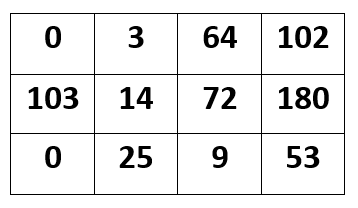As here in our output image, the brightness is decreased by 20 and at the location (0, 0), the pixel value equals 0 but earlier this value was 12. Because subtraction of 20 from 12 will give a negative pixel value (which can not be possible). So, the image pixel value is set to 0.

My Personal Notes arrow_drop_up